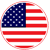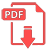# Home | Mathematics | Geometry(Planimetry)

## Ellipse

An ellipse is a curve that is the locus of all points in the plane the sum of whose distances r1 and r2 from two fixed points F1 and F2 (the foci) separated by a distance of 2c is a given positive constant 2a).
This results in the two-center bipolar coordinate equation:

r1+r2=2a,

where a is the semimajor axis and the origin of the coordinate system is at one of the foci. The corresponding parameter b is known as the semiminor axis.English